# bwlabel search order

I've received several questions over the past months about the search order for the function bwlabel and whether it can be changed. Today's post discusses the search-order issue, how useful it might or might not be to change it, and how to use regionprops to reorder labeled objects.

First, though, a brief review of bwlabel: It takes a binary image, bw, and produces a label matrix, L. The elements of L are integer values greater than or equal to 0. The elements equal to 0 are the background. The elements equal to 1 make up one object, the elements equal to 2 make up a second object, and so on. Here's a small example.

bw = false(5, 5);
bw(1:2, 1:2) = true;
bw(4:5, 1:2) = true;
bw(1:2, 4:5) = true;
bw(4:5, 4:5) = true
bw =

1     1     0     1     1
1     1     0     1     1
0     0     0     0     0
1     1     0     1     1
1     1     0     1     1


L = bwlabel(bw)
L =

1     1     0     3     3
1     1     0     3     3
0     0     0     0     0
2     2     0     4     4
2     2     0     4     4



So bwlabel found four connected groups of foreground pixels in bw. Since bwlabel scans the pixels of bw of column order, it finds the object in the lower-left corner second, which is why those pixels are labeled with a "2" in L.

Sometimes people ask if bwlabel can be made to search along the rows instead of along the columns, because they want the object in the upper right to be found second.

The search order for bwlabel is determined by the internal memory storage order of elements in MATLAB. Searching along rows would substantially slow the function down. However, it is possible, with the assistance of regionprops, to efficiently postprocess the output of bwlabel so that objects are ordered however you wish.

Before I show that technique, though, let me show an example of how ordering objects in two dimensions is not always straightforward, no matter which way you search.

Here's a snippet of a scanned text image we've look at before:

url = 'https://blogs.mathworks.com/images/steve/186/scanned_page.png';
bw = ~bw(1107:1194, 17:135);
imshow(bw)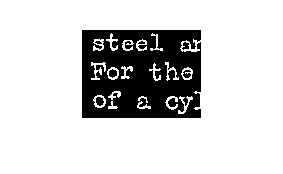One might expect that bwlabel would label the upper-left corner "s" as the first object found. But that doesn't happen.

L = bwlabel(bw);

% Show the object labeled first.
imshow(L == 1)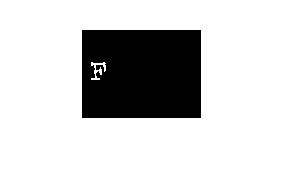Why is the F found first? We can see why if we zoom in on the upper-left corner of the image.

set(gcf, 'Position', [100 100 400 300])
imshow(bw(1:50, 1:20), 'InitialMag', 'fit')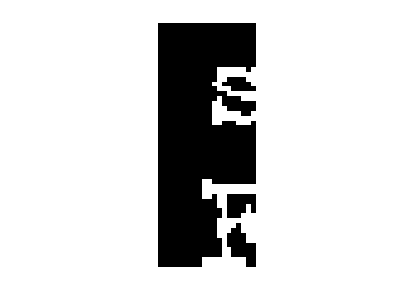The serif on the upper-left of the "F" extends to the left of the letter "s." Therefore, the columnwise scan performed by bwlabel finds the "F" first.

We could force a row-wise labeling search by transposing the binary image and then the output of bwlabel, like this:

L = bwlabel(bw')';

But another surprise awaits; the first object found still isn't the "s"!

imshow(L == 1)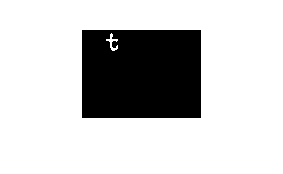The "t" is found first, because the vertical stem of the "t" extends higher than the top of the "s" character.

Also, bwlabel will merge two or more initially assigned labels if it discovers along the way that two or more apparently separate objects are actually connected. This can change the labeling order in unexpected ways.

With those cautionary notes in mind, let me show you how to postprocess the output of bwlabel to sort objects as desired.

First, let's use regionprops to get some object measurements that might be useful for object ordering.

s = regionprops(L, 'BoundingBox', 'Extrema', 'Centroid');

We could sort the objects from left to right according to the left edge of the bounding box.

boxes = cat(1, s.BoundingBox);
left_edge = boxes(:,1);
[sorted, sort_order] = sort(left_edge);
s2 = s(sort_order);

Let's visualize that sort order.

I = im2uint8(bw);
I(~bw) = 200;
I(bw) = 240;
set(gcf, 'Position', [100 100 400 300]);
imshow(I, 'InitialMag', 'fit')
hold on
for k = 1:numel(s2)
centroid = s2(k).Centroid;
text(centroid(1), centroid(2), sprintf('%d', k));
end
hold off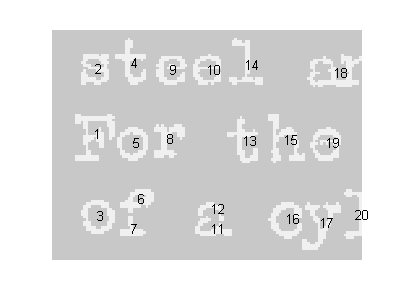To sort according to a row-wise search order, sort lexicographically (using sortrows instead of sort) on the left-most top extremum of each object. This extremum is the first row of the 'Extrema' output from regionprops.

extrema = cat(1, s.Extrema);
left_most_top = extrema(1:8:end, :);
[sorted, sort_order] = sortrows(fliplr(left_most_top));
s2 = s(sort_order);
imshow(I, 'InitialMag', 'fit')
hold on
for k = 1:numel(s2)
centroid = s2(k).Centroid;
text(centroid(1), centroid(2), sprintf('%d', k));
end
hold off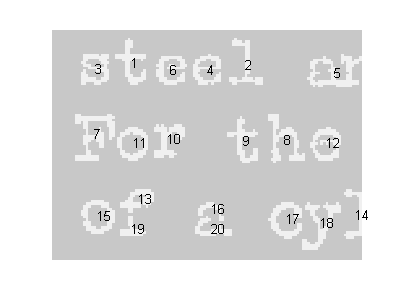Notice how the characters still aren't well-ordered from left to right. That's because the taller ones get sorted earlier. Let's try sorting first on the bottom of each character instead of the top, and quantizing the bottom coordinate. The left-most bottom extremum is the 6th row in 'Extrema' measurement.

left_most_bottom = extrema(6:8:end, :);
left = left_most_bottom(:, 1);
bottom = left_most_bottom(:, 2);
% quantize the bottom coordinate
bottom = 6 * round(bottom / 6);
[sorted, sort_order] = sortrows([bottom left]);
s2 = s(sort_order);
imshow(I, 'InitialMag', 'fit')
hold on
for k = 1:numel(s2)
centroid = s2(k).Centroid;
text(centroid(1), centroid(2), sprintf('%d', k));
end
hold off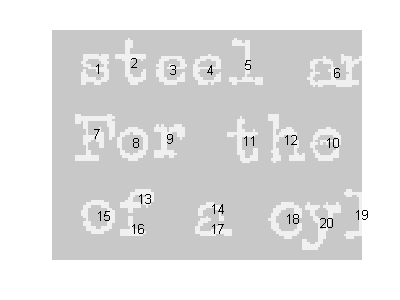That's somewhat better, but to get further I imagine that some higher-level analysis of the structure of text lines in the image will be necessary.

Notice that I did not modify the label matrix, L, in these postprocessing steps. Next time I'll show how to relabel L.

Published with MATLAB® 7.6

|